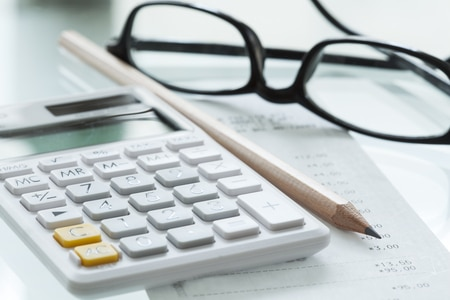# Effective Rate of Interest CalculationSee Also:
What is Compound Interest
When is Interest Rate Not an Important in Selecting a Loan?
Nominal Interest Rate
Interest Rate Swaps
Fixed Interest Rate vs Floating Interest Rate

# Effective Rate of Interest Calculation

An effective rate of interest calculation is the actual cost of a loan. It is the total amount of interest paid on a loan, expressed as a percentage of the principal. Effective annual interest rates incorporate the effects of compounding.

## Effective Annual Rate Formula

Effective annual interest rates are calculated in the two following ways:

1. Effective Rate = Total Interest Paid / Principal Amount

2. Effective Rate = (1 + i / n)n – 1

(Where i is the nominal rate and n is the number of compounding periods per year.)

For example, using the first formula, if the starting principal amount is \$1,000 and the total interest paid over the course of the year is \$104.70, then the effective interest rate is 10.47%. So, look at the following calculation:

.1047 = 104.7 / 1000

Using the second formula, if the starting principal amount is \$1,000, the nominal annual interest rate is 10%, and the rate is compounded monthly, then the effective annual rate is 10.47%. Look at the following calculation:

.1047 = (1 + .10/12)12 – 1

If you want to overcome obstacles and prepare how your company is going to react to external factors, then download your free External Analysis whitepaper.